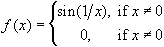#Interactive Real Analysis

Next | Previous | Glossary | Map

## 6.3. Discontinuous Functions

### Examples 6.3.4(c):

Prove that f(x) has a discontinuity of second kind at x = 0This function is more complicated. Consider the sequence xn = 1 / (2 n). As n goes to infinity, the sequence converges to zero from the right. But f( xn) = sin(2 n) = 0 for all k. On the other hand, consider the sequence xn = 2 / ( (2n+1)). Again, the sequence converges to zero from the right as n goes to infinity. But this time f( xn) = sin( (2n+1)/ 2) which alternates between +1 and -1. Hence, this limit does not exist. Therefore, the limit of f(x) as x approaches zero from the right does not exist.

Since f(x) is an odd function, the same argument shows that the limit of f(x) as x approaches zero from the left does not exist.

Therefore, the function has an essential discontinuity at x = 0.

Next | Previous | Glossary | Map